# Heat Transfer in a Semi-Transparent Parallelogram Shaped Medium

Heat Transfer in a Semi-Transparent Parallelogram Shaped Medium

Myriam Lazard

Département FTC, CNRS-Université de Poitiers-ENSMA, UPR 3346, ENSI Poitiers, 2 rue Pierre Brousse, TSA 41105, 86073 POITIERS Cedex, FRANCE

Corresponding Author Email:
myriam.lazard@univ-poitiers.fr
Page:
S420-S424
|
DOI:
https://doi.org/10.18280/ijht.34S232
|
Accepted:
|
Published:
31 October 2016
| Citation

OPEN ACCESS

Abstract:

The coupled conductive radiative heat transfer is investigated within a semi-transparent medium. The two dimensional case of a parallelogram is considered. The specificity of the approach proposed here consists in expressing the divergence term of the radiative heat flux that appears from the temperature. In that way, it remains only one differential equation to solve numerically. Compared to classical approaches, the equation is certainly more complicated but the method is more efficient because the problem becomes uncoupled. Results are presented for different parallelograms more or less flattened.

Keywords:

1. Introduction

Within a semi-transparent medium, both conduction and radiation occur . The first step is usually to deal with the radiative heat transfer itself  and then to investigate the coupled conductive-radiative heat transfer [3-6]. Many authors have investigated this topic in order to predict the coupled heat transfer within these media which are widely used in industry or aerospace such as float glasses or foams. As soon as the geometry is complex, no analytical solution could be found anymore and it is then necessary to perform numerical simulations based for instance on finite element methods [2-8] or even hybrid finite volume/finite element discretization method  and also with neural-finite difference method [10-11] with experimental validation .

Then meshless methods have emerged [12-16]: some are based on local Petrov-Galerkin approach  and usually the moving least squares approximation is used to construct the shape function. Others are based on the natural element method using natural neighbor (Sibson) interpolation or Laplace interpolation [13-15].

One can also notice that non Fourier conduction is analyzed in a differentially heated two dimensional cavity . Recently Galerkin method is still used to solve the combined radiative and conductive heat transfer  whereas lattice Boltzmann is also considered with a finite volume method .

Mainly the case of two dimensional rectangular media is considered. Few studies deal with parallelogram media. The radiative heat transfer was investigated in a parallelogram shaped cavity  but there is no coupling with conduction. Then it is interesting to apply the method, already developed for a rectangular semitransparent medium [21-22], for a parallelogram semitransparent medium.

After the mathematical formulation of the conductive radiative heat transfer, results of the simulations in terms of temperature fields are presented.

2. Physical Model and Mathematical Formulation

2.1 Governing equations

The coupled conductive and radiative heat transfer involves the heat balance equation and the radiative transfer equation which describe the coupled evolution of the temperature T and the intensity L within the semitransparent material.

For a semi-transparent medium, the heat flux is then composed by a conductive component and a radiative component. The general formulation of the heat transfer equation is:

$\rho C_{p}\left(\frac{\partial T}{\partial t}+\overrightarrow{\mathrm{v}} \cdot \vec{\nabla} T\right)=-\vec{\nabla} \cdot\left(\vec{\phi}_{c}+\vec{\phi}_{r}\right)+P$(1)

In the case of a solid medium, there is no convective transport. P represents all the source terms and all the volumic heat sink (other contribution than radiation).

The conductive heat flux is given by the Fourier law:

$\vec{\phi}_{c}=-\lambda \cdot \operatorname{grad}(T)$(2)

The radiative heat flux density is expressed through the intensity:

$\vec{\phi}_{r}(r, t)=\int_{0}^{\infty} \int_{4 \pi} L_{v}^{\prime}(r, \Delta) \vec{n} . \vec{u} d \Omega d v$(3)

The radiative contribution is taken into account via a source term:

$P_{r}=-\operatorname{div}\left(\vec{\phi}_{r}\right)$(4)

That leads to the following equation (in the case of no internal sources P = 0):

$\lambda \Delta T+P_{r}=\rho C_{p} \frac{\partial T}{\partial t}$(5)

The radiative heat flux can be evaluated via the intensity differential equation well known as the radiative transfer equation (RTE):

$\frac{d L^{\prime}}{d s}=-K L^{\prime}(s)+K L_{0}(T)$(6)

This equation represents the intensity variation due to an energy loss by absorption and an energy gain due to the media reemission (emission blackbody law that depends on the temperature of the media at the considered point).

2.2 Expressions of the radiative quantities

2.2.1 The divergence of the heat flux

In the following sections the optical properties of the medium are assumed to be wavelength independent (gray medium) in order to simplify the writing. The extension to the case of a non grey medium raises no numerical difficulties.

The radiative heat flux at a point with s the curvilinear abscissa is given by:

$\vec{q}_{r}(s)=\int_{4 \pi} L^{\prime}(s) \cdot \vec{s} d \Omega$(7)

Thanks to Eq(6), the radiative heat flux divergence could be written as follow:

$\operatorname{div}\left(\vec{q}_{r}\right)=\int_{4 \pi} \frac{d L^{\prime}}{d s} d \Omega=-K \int_{4 \pi} L^{\prime} d \Omega+K \int_{4 \pi} L_{0}(T) d \Omega$(8)

Finally, the radiative heat flux divergence is given by the following equation:

$\operatorname{div}\left(\vec{q}_{r}\right)=-4 \pi K \overline{L}+4 \pi K L_{0}=4 \pi K\left(L_{0}-\overline{L}\right)$(9)

with

$\overline{L}=\frac{1}{4 \pi} \int_{4 \pi} L^{\prime} d \Omega$ and $L_{0}=\frac{n^{2} \sigma T^{4}}{\pi}$(10)

The blackbody intensity and the average intensity are expressed as a temperature function. As radiation is a transfer without any contact, the average intensity at a given point is a function of all the temperatures of the medium and of the frontiers. The intensity depends on the medium’s geometry.

2.2.2 The radiative heat flux and the average intensity

In the general case of three-dimensional problems, the expressions of the radiative heat flux and the average intensity are given by:

$\vec{q}_{r}(\vec{r})=\int_{A} L^{\prime}\left(r_{0}\right)\left[\vec{n} \cdot\left(\vec{r}-\vec{r}_{0}\right)\right] \frac{\vec{r}-\vec{r}_{0}}{\left|\vec{r}-\vec{r}_{0}\right|^{4}} \exp \left(-K\left|\vec{r}-\vec{r}_{0}\right|\right) d A$$+K \int_{V} L^{\prime}\left(r^{*}\right) \frac{\vec{r}-\vec{r}^{*}}{\left|\vec{r}-\vec{r}^{*}\right|^{3}} \exp \left(-K\left|\vec{r}-\vec{r}^{*}\right|\right) d V^{*}$(11)

\begin{aligned} \overline{L}(\vec{r})=& \frac{1}{4 \pi} \int_{A} L^{\prime}\left(r_{0}\right) \frac{\vec{n} \cdot\left(\vec{r}-\vec{r}_{0}\right)}{\left|\vec{r}-\vec{r}_{0}\right|^{3}} \exp \left(-K\left|\vec{r}-\vec{r}_{0}\right|\right) d A \\ &+\frac{K}{4 \pi} \int_{V} L^{\prime}\left(r^{*}\right) \frac{\exp \left(-K\left|\vec{r}-\vec{r}^{*}\right|\right)}{|\vec{r}-\vec{r}|^{2}} d V^{*} \end{aligned}(12)

K represents the average absorption coefficient for a semi-transparent medium with a surface A and with a volume V.

The two vectors that is to say the radiative heat flux and the average intensity at a point M (x, y, z) of the volume V, spotted towards the reference point O and the radius vector $\vec{r}$. A point M0 (x0, y0, z0) of the surface A is spotted towards $\vec{r}_{0}$. The vectors $\vec{r}_{0}, \vec{r}$ et $\vec{r}^{*}$ permit to locate the position of the surface and the volumes dA, dV et dV *.

One can clearly see in the Eq(12) that there are two contributions to the radiative heat flux and the average intensity: a surface integral and a volume integral.

3. Numerical Resolution and Results

3.1 Numerical resolution

The differential equation could be written:

$\lambda \cdot \operatorname{div}(\overrightarrow{\operatorname{grad}}(T))-\operatorname{div}\left(\vec{q}_{r}\right)=\rho C_{p} \frac{\partial T}{\partial t}$(13)

with

$\operatorname{div}\left(\vec{q}_{r}\right)=4 K n^{2} \sigma T^{4}(x, y)-4 \pi K \overline{L}$(14)

The differential equation is nonlinear in temperature owing to the term with the divergence of the radiative heat flux. It could be solved for each time step with an iterative method. To initialize the procedure, the solution of the purely conductive problem could be used. It is more interesting and judicious to use the solution of the coupled problem after the linearization of the radiative component

3.2 Validations

First of all, simulations are made in test cases such as one dimensional slab in steady then transient state. The simulations agree with others results obtained by semi-analytical methods or classical methods . For instance in the Figure 1, the temperature fields are plotted in the case of the flash method (this method consists in measuring the temperature and especially the rear face transient temperature of a sample when its front face absorbs a heat pulse)

## figure_1.png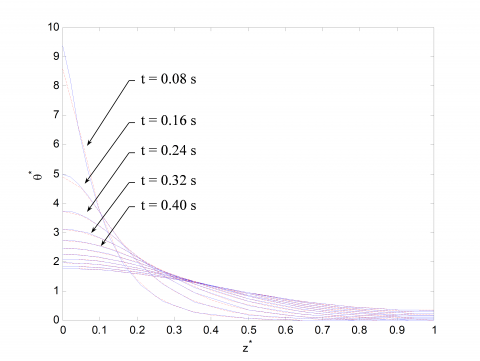Figure 1. Temperature fields at different times

Then the case of a two dimensional rectangular semi-transparent medium is considered (see Figure 2). The emissivity of each wall is equal to one. The thermal conductivity of the medium is equal to 1 W.m-1. K-1. The coefficient of absorption is equal to 1 m-1. The temperature at the bottom is imposed at 1000 K.

3.3 Simulations in the case of a parallelogram

Numerical simulations are then performed in the two dimensional case of a parallelogram. The same value for the thermophysical parameters are considered. The results obtained are presented on the following figures.

## figure_2.jpg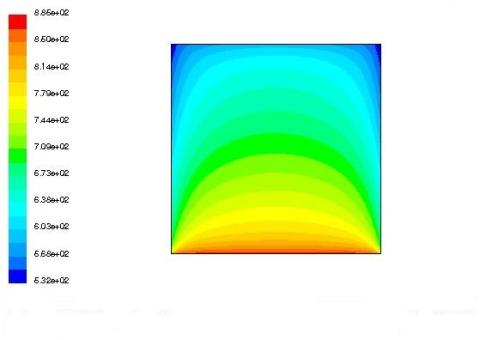Figure 2. Temperature profile (angle 90°)

## figure_3.jpg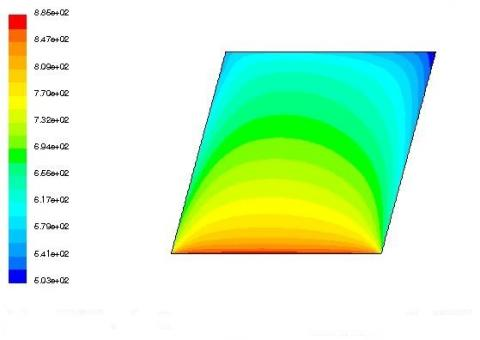Figure 3. Temperature profile (angle 75°)

## figure_4.jpg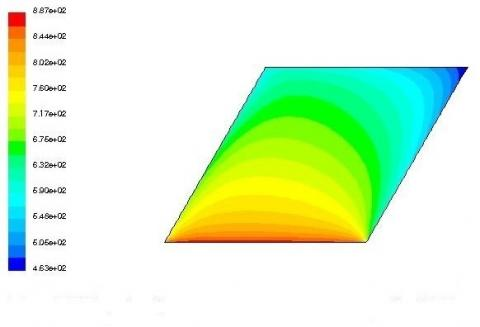Figure 4. Temperature profile (angle 60°)

## figure_5.jpg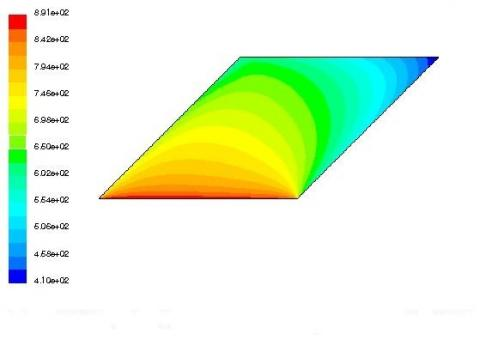Figure 5. Temperature profile (angle 45°)

## figure_6.jpg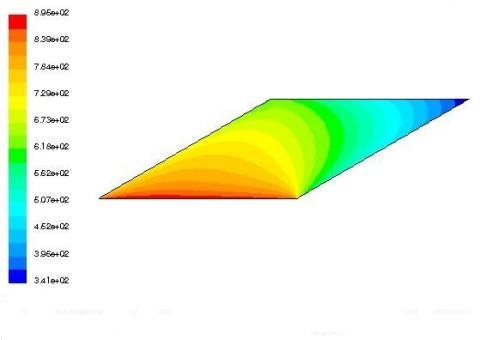Figure 6. Temperature profile (angle 30°)

## figure_7.jpg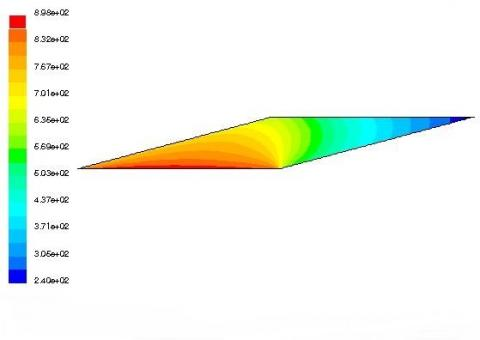Figure 7. Temperature profile (angle 15°)

Whatever the value of the angle, it is possible to obtain the temperature field whereas in other studies, authors encountered difficulties as soon as the angle is smaller than 30°.

4. Conclusions

After a synthetic state of art and the main equations of the thermal behaviour of a semi-transparent medium, numerical simulations are carefully performed in order to solve the energy balance and the radiative transfer equation. The specificity of the approach proposed here consists in expressing the divergence term of the radiative heat flux that appears from the temperature. In that way, it remains only one differential equation to solve numerically. The aim is to obtain the temperature within a participating parallelogram shaped medium. The main difficulty could have occur for the mesh when the angle of the parallelogram becomes very sharp. Nevertheless, temperature fields could be obtained.

Nomenclature
 A surface m2 CP specific heat, J. Kg-1. K-1 K absorption coefficient m-1 L’ radiant intensity W/sr n refractive index P source terms and volumetric heat sink q heat flux W r norm of the radius vector s curvilinear abscissa t time s T temperature K V volume m3 Greek symbols $\alpha$ thermal diffusivity, m2. s-1 $\lambda$ thermal conductivity, W.m-1. K-1 $\phi$ heat flux density, W.m-2 $\rho$ density $\Omega$ solid angle sr Subscripts c conduction r radiation 0 relative to the blackbody
References

 R. Viskanta and R. J. Grosh, “Heat transfer by simultaneous conduction and radiation in an absorbing medium,” J Heat Transfer, vol. 84, pp. 63–72, 1962.

 M. Razzaque, D. E. Klein and J. R. Howell, “Finite element solution of radiative heat transfer in a two-dimensional rectangular enclosure with gray participating media,” J Heat Transfer, vol. 105, pp. 933–944, 1983.

 M. Razzaque, J. R. Howell and D. E. Klein, “Coupled radiative and conductive heat transfer in a two dimensional rectangular enclosure with gray participating media using finite elements,” J Heat Transfer, vol. 106, pp. 613–619, 1984.

 W.W. Yuen and E. E. Takara, “Analysis of combined conductive-radiative heat transfer in a two-dimensional rectangular enclosure with a gray medium,” J Heat Transfer, vol. 110, pp. 468–474, 1988.

 M. Nourohalli et al., “Numerical prediction of coupled conduction, convection and radiation heat transfer,” International Journal of Heat and Technology, vol. 27, pp. 87–94, 2009.

 M. Al_Khawaja, “Numerical study of combined natural convection, conduction and surface radiation heat transfer in open top, side vented cavities,” International Journal of Heat and Technology, vol. 26, pp. 123–126, 2008.

 J. Braun and M. Sambridge, “A numerical method for solving partial differential equations on highly irregular evolving grids,” Nature, vol. 376, pp. 655–660, 1995.

 M. Ben Salah, et al., “Control volume finite element method for radiation,” J. of Quantitative Spectroscopy and Radiative Transfer, vol. 92, pp. 9-30, 2005. DOI: 10.1016/j.jqsrt.2004.07.008.

 P. J. Coehlo, “A hybrid finite volume/finite element discretization method for the solution of the radiative heat transfer equation,” J. of Quantitative Spectroscopy and Radiative Transfer, vol. 93, pp. 89-101, 2005. DOI: 10.1016/j.jqsrt.2004.08.014.

 J. F. Luo et al, “Conduction and radiation in a rectangular isotropic scattering medium with black surfaces by the RTNAM,” Int. J. Heat and Mass Transfer, vol. 52, pp. 5064-5071, October 2009. DOI: 10.1016/j.ijheatmasstransfer.2009.04.032.

 R. K. Mallik, S. K. Mahapatra and A. Sarkar, “Neural-finite difference method (NFDM) in development of improved differential approximation (IDA) and its application for coupled conduction and radiation heat transfer in a square enclosure: an experimental validation,” Int. J. Heat and Mass Transfer, vol. 52, pp. 504-515, January 2009. DOI: 10.1016/j.ijheatmasstransfer.2008.04.073.

 L. H. Liu and J. Y. Tan, “Meshless local Petrov-Galerkin approach for coupled radiative and conductive heat transfer,” Int J Thermal Sciences, vol. 46, no. 7, pp. 672–681, July 2007. DOI: 10.1016/j.ijthermalsci.2006.09.005.

 Y. Zhang, H. L. Yi and H. P. Tan, “Natural element method analysis for coupled radiative and conductive heat transfer in a semitransparent medium with irregular geometries,” Int. J. Thermal Sciences, vol. 76, no. 3, pp. 30-42, February 2014. DOI: 10.1016/j.ijthermalsci.2013.08.13.

 Y. Zhang, H. L. Yi and H. P. Tan, “Natural element method analysis for solving radiative transfer with or without conduction in three dimensional complex geometries,” J. of Quantitative Spectroscopy and Radiative Transfer, vol. 129, pp. 118-130, November 2013. DOI: 10.1016/j.jqsrt.2013.06.002.

 Y. Zhang, H. L. Yi and H. P. Tan, “Natural element method analysis for radiative heat transfer in two dimensional semitransparent medium,” Int. J. Heat and Mass Transfer, vol. 56, pp. 411-423, January 2013. DOI: 10.1016/j.ijheatmasstransfer.2012.09.051.

 C. A. Wang, H. Sadat, M. Lazard and V. Le Dez, “Meshless method for solving multidimensional transient radiative transfer,” 7th International Symposium on Radiative Transfer, Kusadasi, Turkey, 2-8 June 2013, In Begell House, Inc CD-ROM.

 A. Sasmal and S. Mishra, “Analysis of non-Fourier conduction and radiation in a differentially heated 2-D square cavity,” Int. J. Heat and Mass Transfer, vol. 79, pp. 116-125, December 2014. DOI: 10.1016/j.ijheatmasstransfer.2014.08.010.

 M. Ghattassi, et al., “Galerkin method for solving combined radiative and conductive heat transfer,” Int J Thermal Sciences, vol. 102, pp. 122–136, April 2016. DOI: 10.1016/j.ijthermalsci.2015.10.011.

 Y. Sun and X. Zhang, “Analysis of transient conduction and radiation problems using lattice Bolzmann and finite volume methods,” Int. J. Heat and Mass Transfer, vol. 97, pp. 611-617, June 2016. DOI: 10.1016/j.ijheatmasstransfer.2012.09.051.

 V. Le Dez and H. Sadat, “Radiative heat transfer in a parallelogram shaped cavity,” Int. Communications in Heat and Mass Transfer, vol. 68, pp. 137-149, November 2015. DOI: 10.1016/j.icheatmasstransfer.2015.08.028.

 M. Lazard, “Simulations de rayonnement par la méthode des éléments finis,” 5ème journées d’études en rayonnement thermique, Nancy, France, 8-9 décembre 2011.

 M. Lazard, “Heat transfer by conduction and radiation in a two-dimensional semi-transparent medium using the finite element method,” Computer and Experimental Simulations in Engineering and Science, ISSN: 1791-3829, 2008.